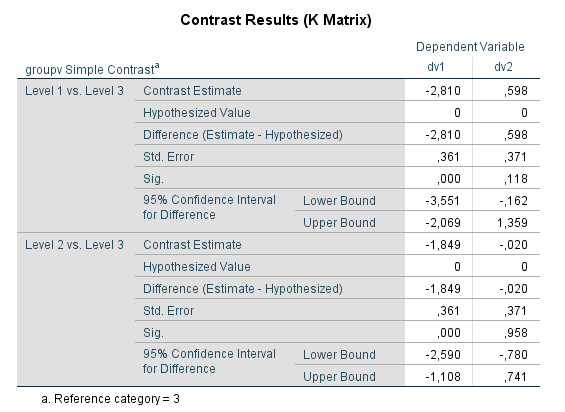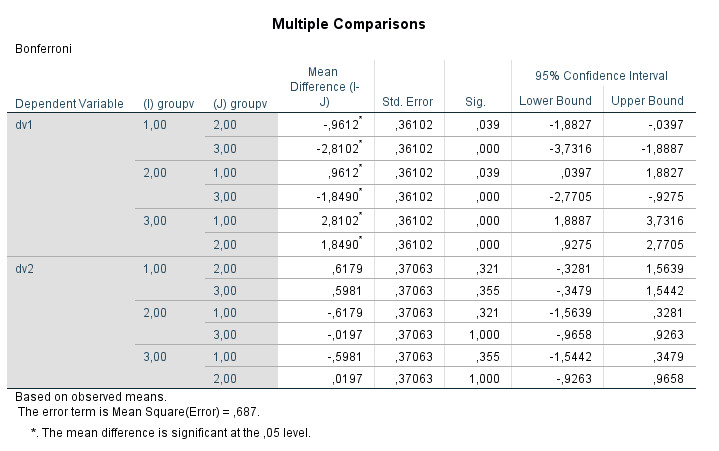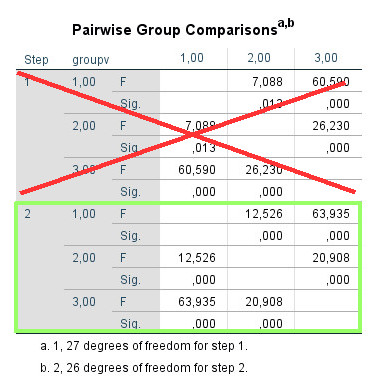﻿ MANOVA: Planned multivariate contrasts with SPSS

# Contrasts for MANOVA Planned multivariate comparisons with SPSS

Arndt Regorz, Dipl. Kfm. & M.Sc. Psychologie, 09/13/2019

If you compare three or more groups on two or more dependent variables a significant MANOVA-result is not enough, in most cases. You need multivariate (!) group comparisons (contrasts) in order to assess which groups differ.

But this functionality is very well hidden in SPSS (Version 25) since the MANOVA window only contains the option for univariate contrasts.

This tutorial will show you how to get to multivariate contrasts for your one-way MANOVA with three or more groups.

## 1. Video-Tutorial

(Note: When you click on this video you are using a service offered by YouTube.)

## 2. The problem

MANOVA, i.e. multivariate analysis of variance, is used to compare two or more groups on two or more metric dependent variables.

If we have only two groups it is enough to look at the results of the MANOVA; if those are significant we know that the two groups differ on a linear combination of the dependent variables.

But what if we have more than two groups? Then a significant MANOVA is but the first step. The null hypothesis for MANOVA is that there exist no differences between the groups on a linear combination of the dependent variables. Therefore a significant MANOVA tells us that at least two groups differ on a combination of the dependent variables, but with more than two groups we do not know which groups differ. It is the same problem you have when doing (univariate) ANOVA with three and more groups. For that we need planned comparisons (= contrasts).

## 3. The theoretical solution: multivariate contrasts

So we need group comparisons, i.e. planned contrasts. We want to compare specific groups on a linear combination of the dependent variables: multivariate contrasts. As univariate contrasts are group comparisons for a significant ANOVA (group comparisons for one dependent variable) multivariate contrasts are group comparisons for a significant MANOVA (group comparison for a linear combination of two or more dependent variables).

The problem is: This functionality is very well hidden in SPSS (Version 25).

## 4. Carrying out multivariate contrasts from the SPSS dialog box?

If you look for contrasts in the MANOVA dialog box you will find a contrast option:
Analyze->General Linear Model->Multivariate
And then on the right side of the window there is a button for “Contrasts”

It looks good, but if you use it, unfortunately, you get something like this (depending on the type of contrast you choose):And if you use “Post hoc” you get something like this (depending on the type of post hoc test):In both cases you do get group comparisons. But the group comparisons are univariate, i.e. group comparisons for one dependent variable at a time. That would be the appropriate thing to do for a significant (univariate) ANOVA. But if you had wanted to do univariate tests you would have used two or more ANOVAs instead of a MANOVA in the first place.

## 5. Multivariate contrasts: By syntax only

Currently (SPSS Version 25) you can carry out multivariate contrasts by syntax only. And in a very indirect form, at that. Because you have to run a linear discriminant analysis and not MANOVA for that (IBM, n.d.). Then the program tests the Mahalanobis distance between each pair of levels of the group variable.

How is it possible that you get multivariate contrasts for MANOVA by running a linear discriminant analysis? Discriminant analysis and MANOVA are technically more or less the same. With the same data you get identical test statistics (Wilk's Lambda) and p-values. Those are just different perspectives on the same problem.

With MANOVA we want to explain values on two or more metric dependent variables with the membership in different groups. With discriminant analysis we want to predict the membership in different groups with the values of two or more metric variables.

To make it even more complicated, you have to call the linear discriminant analysis with stepwise inclusion of predictors (= the dependent variables for the MANOVA). That does not make sense if we want to do contrasts for MANOVA but we have to do it anyway and then only look at the last step of the comparisons where all predictors (= dependent variables for the MANOVA) are included in the model.

## 6. Syntax for MANOVA with planned multivariate contrasts

Here is a syntax example for MANOVA and contrasts (multivariate and univariate), based on IBM (n.d.) with some adjustment to the GLM parameters. The two dependent variables for the MANOVA (and thus predictors for the discriminant analysis) are dv1 and dv2, the grouping variable is called groupv in this example.

GLM dv1 dv2 BY groupv
/METHOD=SSTYPE(3)
/INTERCEPT=INCLUDE
/PRINT=DESCRIPTIVE ETASQ HOMOGENEITY
/CRITERIA=ALPHA(.05)
/DESIGN= groupv.

DISCRIMINANT GROUPS = groupv(1 3)
/VARIABLES= dv1 dv2
/METHOD = WILKS
/FIN=0
/FOUT=0
/STATISTICS = FPAIR .

Probably, you will be getting this warning: “Since ANALYSIS was omitted for the first analysis, all variables on the VARIABLES list will be entered at level 2 when METHOD = DIRECT and at level 1 when a stepwise METHOD.”
Since the parameter "ANALYSIS" was omitted in the syntax on the IBM help page, too, I do not think this really matters.

## 7. Interpretation of key results for contrasts (SPSS Output)

Here is the key table from the SPSS Output with regards to group comparisons:Please remember to interpret the results of the last step only, in this case step 2:In this table we can find the following multivariate group differences:
Group1-Group2: F(2, 26) = 12.53, p < .001
Group1-Group3: F(2, 26) = 63.94, p < .001
Group2-Group3: F(2, 26) = 20.91, p < .001

If you use more than one comparison for one of your hypotheses you have to deal with the problem of multiple testing (e.g. by using Bonferroni correction).

And using discriminant analysis only works for a single factor MANOVA, unfortunately.